Related Articles

# Wine Quality Prediction – Machine Learning

• Last Updated : 07 Sep, 2021

Here we will predict the quality of wine on the basis of giving features. We use the wine quality dataset from Kaggle. This dataset has the fundamental features which are responsible for affecting the quality of the wine. By the use of several Machine learning models, we will predict the quality of the wine. Here we will only deal with the white type wine quality, we use classification techniques to check further the quality of the wine i.e. is it good or bed.

Dataset: here

Attention reader! Don’t stop learning now. Get hold of all the important Machine Learning Concepts with the Machine Learning Foundation Course at a student-friendly price and become industry ready.

Dataset description:

In this dataset, classes are ordered, but it was not balanced. Here, red wine instances are present at a high rate and white wine instances are less than red.

These are the name of Features from the dataset -:

1. type
2. fixed acidity
3. volatile acidity
4. citric acid
5. residual sugar
6. chlorides
7. free sulfur dioxide
8. total sulfur dioxide
9. density
10. pH
11. sulphates
12. alcohol
13. quality

## Python3

 `# import libraries` `import` `pandas as pd``import` `numpy as np``import` `matplotlib.pyplot as plt``import` `seaborn as sb`

Pandas is a useful library in data analysis, Numpy library used for working with arrays, Seaborn and Matplotlib are used in data visualization.

## Python3

 `# loading the data``Dataframe ``=` `pd.read_csv(R``'D:\\xdatasets\winequalityN.csv'``)`

## Python3

 `# show rows and columns``Dataframe.head()`

Output: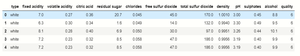## Python3

 `# getting info.``Dataframe.info()`

Output: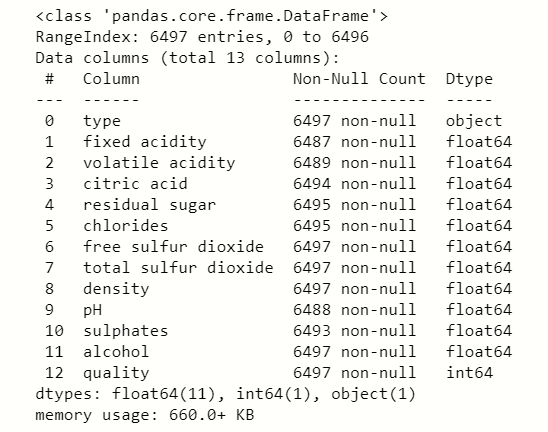## Python3

 `Dataframe.describe()`

Output: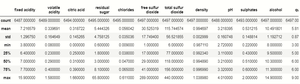## Python3

 `# null value check``Dataframe.isnull().``sum``()`

Output: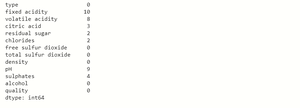## Python

 `# plot pairplot``sb.pairplot(Dataframe)``#show graph``plt.show()`

Output: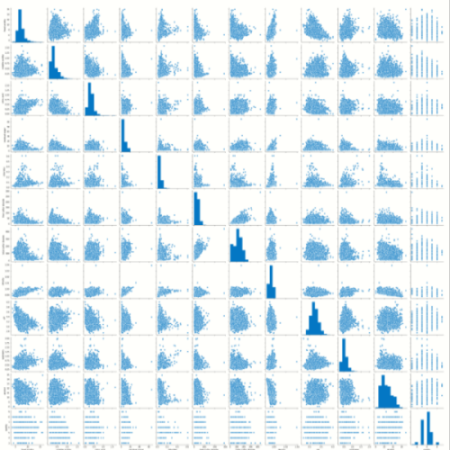## Python3

 `#plot histogram``Dataframe.hist(bins``=``20``,figsize``=``(``10``,``10``))``#plot showing``plt.show()`

Output: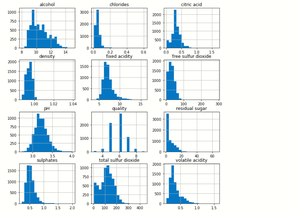## Python3

 `plt.figure(figsize``=``[``15``,``6``])``plt.bar(df[``'quality'``],df[``'alcohol'``])``plt.xlabel(``'quality'``)``plt.ylabel(``'alcohol'``)``plt.show()`

Output: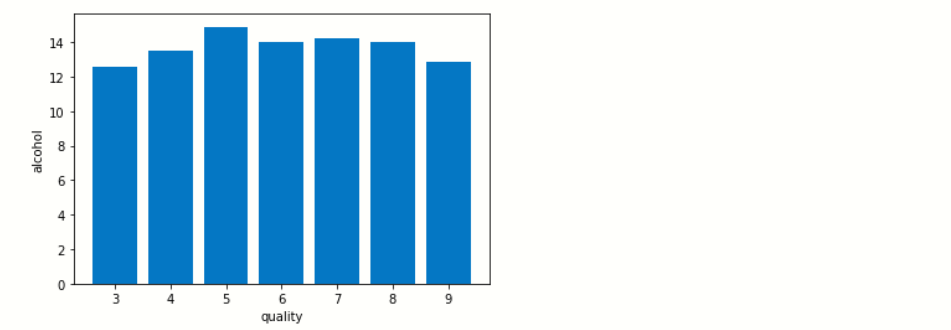s

We check how the quality of wine increases with increase the percent of alcohol in the wine.

### Checking the correlation:

Here we use statistical method which is used to evaluate the strength of bonding of the relationship between two quantitative variables.

## Python3

 `# correlation by visualization``plt.figure(figsize``=``[``18``,``7``])``# plot correlation``sb.heatmap(Dataframe.corr(),annot``=``True``)``plt.show()`

Output: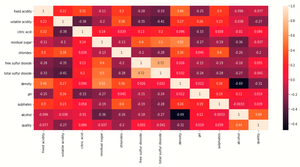From this correlation visualization, we will find which features are correlated with other features. so we will use a python program to find those features.

## Python3

 `colm ``=` `[]``# loop for columns``for` `i ``in` `range``(``len``(Dataframe.corr().keys())):``# loop for rows``  ``for` `j ``in` `range``(j):``    ``if` `abs``(Dataframe.corr().iloc[i,j]) > ``0.7``:``      ``colm ``=` `Dataframe.corr().columns[i]`

By these code we will found that ” total sulfur dioxide ” having a correlation greater than 0.7 values so, we drop this column.

## Python3

 `# drop column``new_df ``=` `Dataframe.drop(``'total sulfur dioxide'``,axis ``=` `1``)`

### Fill null value:

We fill all null values with mean values of specific features and directly update the dataset with update() method.

## Python

 `new_df.update(new_df.fillna(new_df.mean()))`

## Python3

 `# no of categorical columns``cat ``=` `new_df.select_dtypes(include``=``'O'``)``# create dummies of categorical columns``df_dummies ``=` `pd.get_dummies(new_df,drop_first ``=` `True``)``print``(df_dummies)`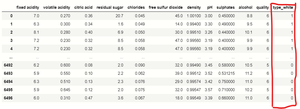We use pandas get_dummies() function which is used for handling categorical columns, in this dataset ‘Type’ feature contain two types Red and white, so get_dummies() function converts this into binary format because the computer didn’t understand object types. As red wine is 1 and white wine is 0.

### Dependent and Independent features:

Basically, we will use classification techniques to fit our model into the dataset for better accuracy, so we will do some fundamental changes in the dependent feature.

## Python3

 `df_dummies[``'best quality'``]``=``[``1` `if` `x>``=``7` `else` `0` `for` `x ``in` `Dataframe.quality]``print``(df_dummies)`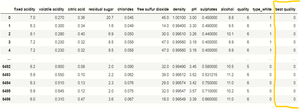We create a program in which if the dependent feature “quality”  values are greater than 7 then it will be considered as 1 and if they are less than 7, then we considered it as 0 and this will be store in new created column “best quality”.

## Python3

 `# import libraries``from` `sklearn.preprocessing ``import` `train_test_split` `# independent variables``x ``=` `df_dummies.drop([``'quality'``,``'best quality'``],axis``=``1``)``# dependent variable``y ``=` `df_dummies[``'best quality'``]` `# creating train test splits``xtrain,xtest,ytrain,ytest ``=` `train_test_split(x,y,test_size``=``0.2``,random_state``=``40``)`

### Normalization of numerical features:

We will use the Normalization technique to scaling our data because in our features data range high, so this technique balances the ranges between 0 and 1.

## Python3

 `# code``# import libraries` `from` `sklearn.preprocessing ``import` `MinMaxScaler``# creating scaler scale var.``norm ``=` `MinMaxScaler()``# fit the scale``norm_fit ``=` `norm.fit(xtrain)``# transformation of training data``scal_xtrain ``=` `norm_fit.transform(xtrain)``# transformation of testing data``scal_xtest ``=` `norm_fit.transform(xtest)``print``(scal_xtrain)`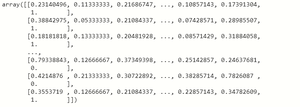After the transformation training and testing data automatic converted into a form of an n-dimensional array.

### Applying the models:

We will apply multiple Regression and Classification models for checking the accuracy score but the RandomForestClassifier gives us the best accuracy as compare to another model, so we use RandomForestClassifier.

## Python3

 `# code``#import libraries``from` `sklearn.ensemble ``import` `RandomForestClassifier` `# for error checking``from` `sklearn.matrics ``import` `mean_squared_error` `from` `sklearn.metrics ``import` `classification_report` `# create model variable``rnd ``=` `RandomForestClassifier()` `# fit the model``fit_rnd ``=` `rnd.fit(new_xtrain,ytrain)` `# checking the accuracy score``rnd_score ``=` `rnd.score(new_xtest,ytest)` `print``(``'score of model is : '``,rnd_score)` `print``(``'.................................'``)` `print``(``'calculating the error'``)` `# checking mean_squared error``MSE ``=` `mean_squared_error(ytest,y_predict)` `# checking root mean squared error``RMSE ``=` `np.sqrt(MSE)` `print``(``'mean squared error is : '``,MSE)` `print``(``'root mean squared error is : '``,RMSE)` `print``(classification_report(ytest,x_predict))`

Output: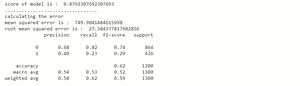The accuracy score of RandomForestClassifier  is 88% and the error rate is also low as compared to other models. This model predicts the quality of white wine with an accuracy of 88%.

### Prediction of values:

Compare the predicted values and our original values to check is our model predicted the true values or not:

## Python3

 `# code``x_predict ``=` `list``(rnd.predict(xtest))``df ``=` `{``'predicted'``:x_predict,``'orignal'``:ytest}``pd.DataFrame(df).head(``10``)`

Output:According to our output, we saw that the original testing values are as much similar to our RandomForestClassifier model predicted values. Here 1 represents the quality greater than 0.7 which is considered in good quality wine and 0 represents the quality below 0.7 which is not considered as a good quality wine.

My Personal Notes arrow_drop_up